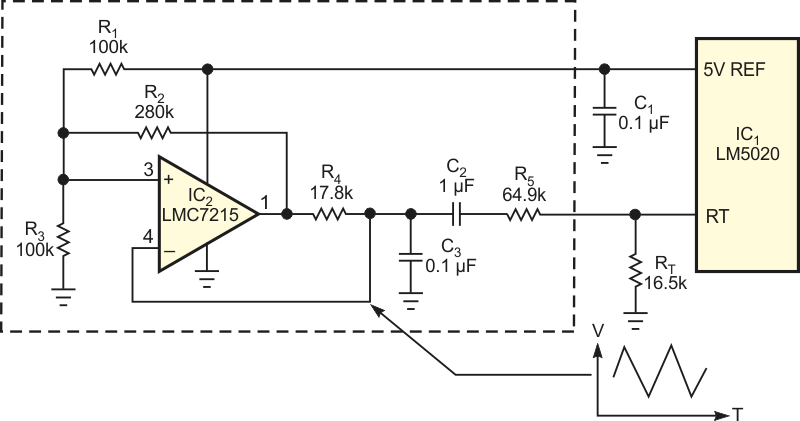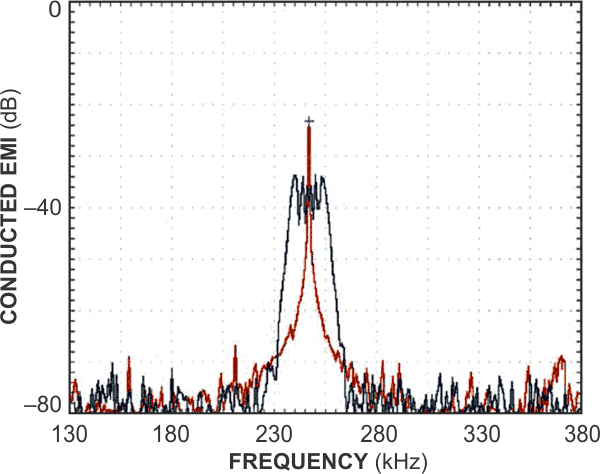# Dither a power converter's operating frequency to reduce peak emissions

## Texas Instruments LM5020 LMC7215

Bob Bell

EDN

Designers of dc/dc switching power converters face the challenge of controlling EMI (electromagnetic-interference) emissions produced during normal operation. If large enough, these emissions conduct through power lines or radiate to other assemblies within a system and can compromise a system's performance. Emission peaks typically occur at the converter's fundamental switching frequency and gradually reduce in amplitude at each higher order harmonic, with most of the emitted energy confining itself to the fundamental and lower order harmonics. Modulating, or dithering, the power converter's operating frequency can reduce the peak emissions by spreading EMI over a band of frequencies.

Most modern PWM controllers use an external resistor to set the operating frequency, which typically increases with decreasing resistor values. For example, the LM5020's internal oscillator delivers a regulated 2 V at its programming pin (RT), and a programming resistor connected to RT sets the current that RT delivers. The oscillator also delivers a proportional current into an internal timing capacitor. The period of the timing capacitor's ramping voltage determines the oscillator's frequency.

The external dithering circuit in Figure 1 comprises a simple stand-alone comparator-based oscillator configured to operate at approximately 800 Hz. The output state of comparator IC2 goes high upon power-up. R1, R2, and R3 set the comparator's positive input, which initially rests at 2.9 V. The voltage at capacitor C3 ramps up toward the positive threshold.Figure 1. A low-frequency triangular-wave oscillator modulates a PWM controller’s oscillator frequency.

When the voltage at the comparator's negative input reaches the positive-threshold voltage, the comparator's output switches low, which also lowers the threshold at the comparator's positive input to 2.1 V. The voltage at capacitor C3 then ramps down toward the new threshold, and, when it reaches the lower threshold voltage, the cycle repeats. The voltage across C3 approximates a triangular wave with a minimum voltage of 2.1 V and a maximum of 2.9 V.

To dither the LM5020 controller's PWM-oscillator base frequency, the triangular wave generated by IC2 modulates the current from the controller's RT pin. Resistor R5 sets the percentage of modulation dither. The right side of R5 is fixed at the RT pin's regulated potential of 2 V, and the low-frequency triangle wave coupled from IC2 through capacitor C2 drives R5's left side. For R5 with a value of 64.9 kΩ, the peak-to-peak current through resistor R5 is approximately 12 µA. With the dither circuit disconnected, the steady-state current that RT sources is approximately 121 µA, and the 12-µA p-p dither current thus represents 10% total modulation.

An LM5020-controlled PWM flyback dc/dc converter, IC1, evaluates the dither circuit's effectiveness. The circuit's fundamental operating frequency is 250 kHz, which the controller's RT resistor sets. The red trace of Figure 2 shows the conducted emissions on the circuit's positive input-power line without the dither circuit in operation. The peak emissions are narrowly confined around the fundamental oscillator frequency of 250 kHz with a measured amplitude at the fundamental frequency of –24 dB.Figure 2. Measured at the dc/dc converter’s input, peak conducted emissions without dither (red trace) decrease by 10 dB with dithering applied (blue trace).

Connecting the dither circuit to the controller's RT input produces the blue trace of Figure 2. Conducted emissions around the fundamental frequency now disperse around the fundamental frequency with maximum amplitude reduced by –34 to +10 dB.

EDN